Скачать презентацию Math 344 Winter 07 Group Theory Part 3

711a83651f91a617841ffeb87422039e.ppt

• Количество слайдов: 21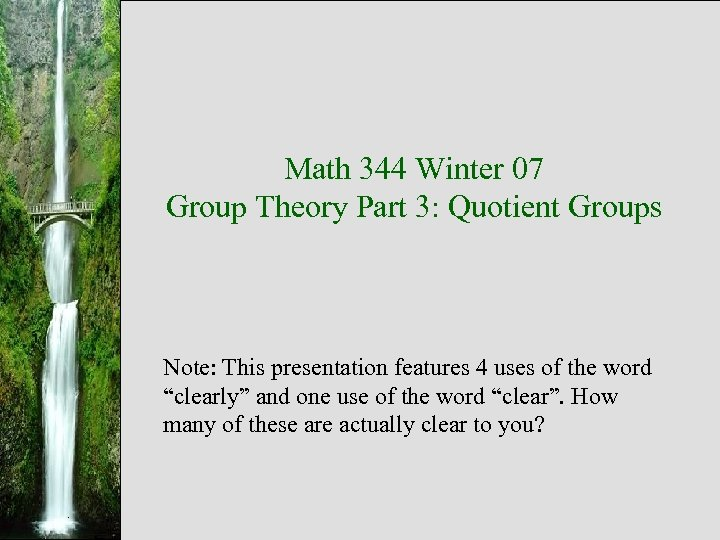Math 344 Winter 07 Group Theory Part 3: Quotient Groups Note: This presentation features 4 uses of the word “clearly” and one use of the word “clear”. How many of these are actually clear to you?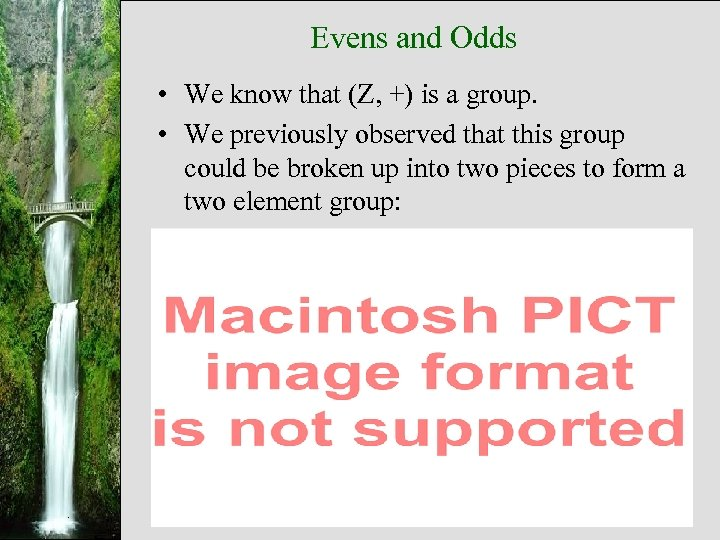Evens and Odds • We know that (Z, +) is a group. • We previously observed that this group could be broken up into two pieces to form a two element group: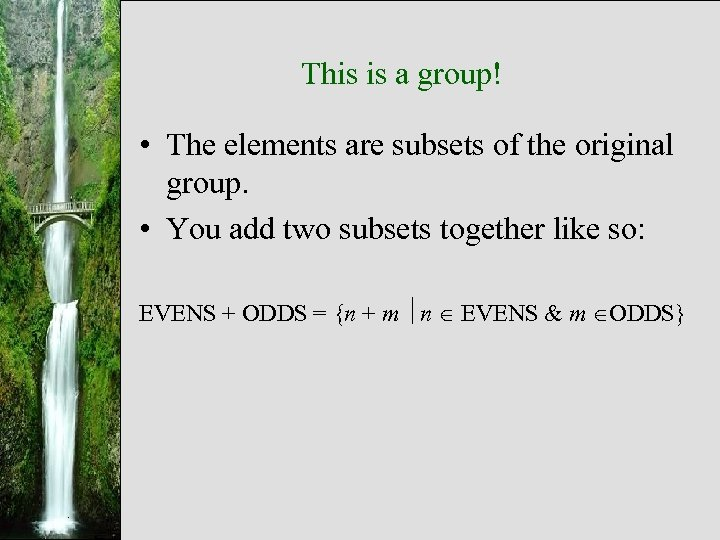This is a group! • The elements are subsets of the original group. • You add two subsets together like so: EVENS + ODDS = {n + m n EVENS & m ODDS}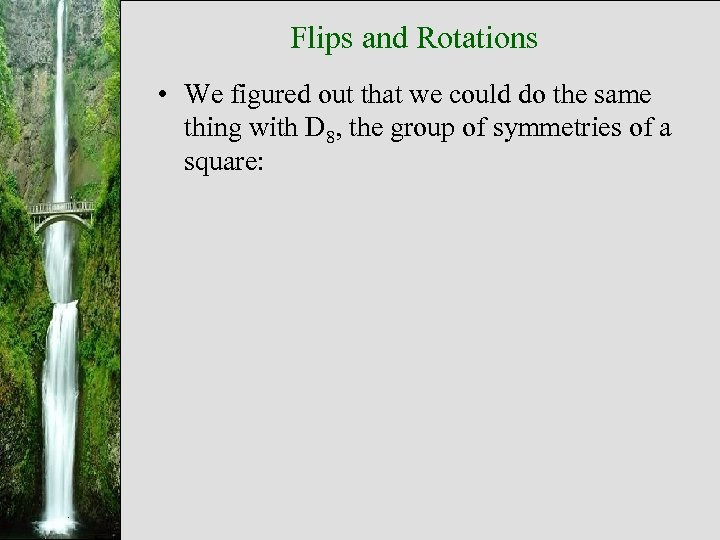Flips and Rotations • We figured out that we could do the same thing with D 8, the group of symmetries of a square: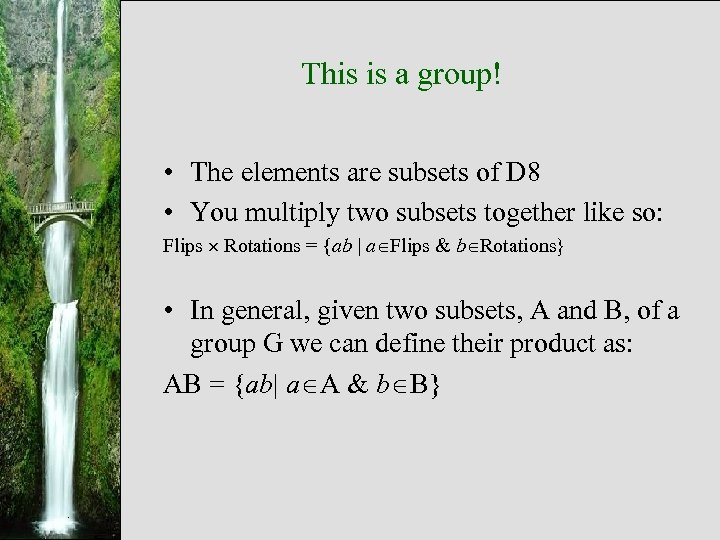This is a group! • The elements are subsets of D 8 • You multiply two subsets together like so: Flips Rotations = {ab a Flips & b Rotations} • In general, given two subsets, A and B, of a group G we can define their product as: AB = {ab a A & b B}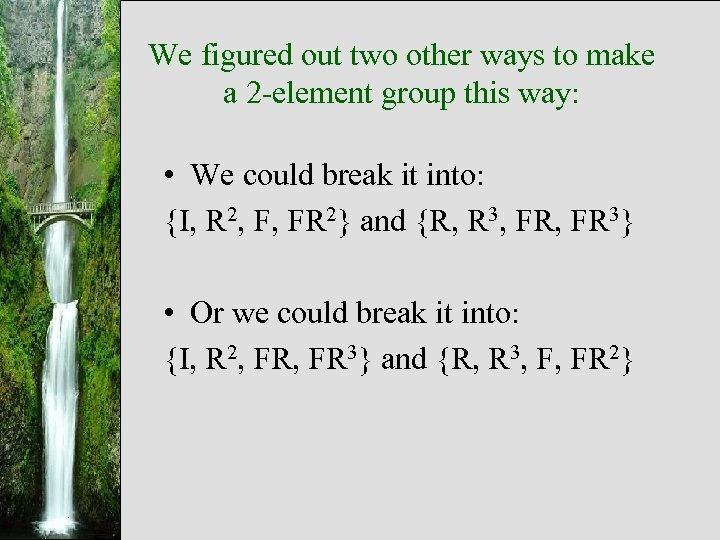We figured out two other ways to make a 2 -element group this way: • We could break it into: {I, R 2, F, FR 2} and {R, R 3, FR 3} • Or we could break it into: {I, R 2, FR 3} and {R, R 3, F, FR 2}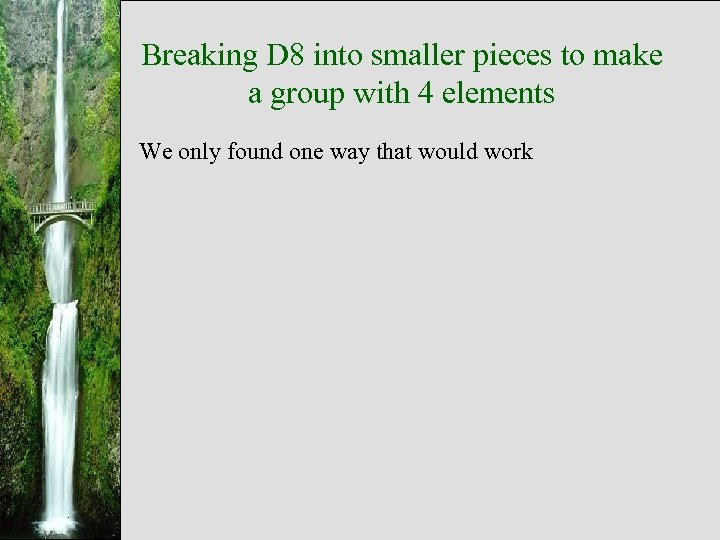Breaking D 8 into smaller pieces to make a group with 4 elements We only found one way that would work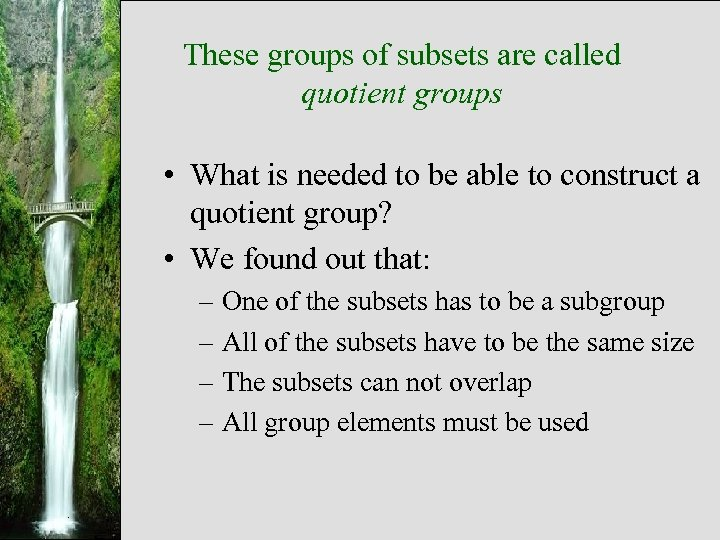These groups of subsets are called quotient groups • What is needed to be able to construct a quotient group? • We found out that: – One of the subsets has to be a subgroup – All of the subsets have to be the same size – The subsets can not overlap – All group elements must be used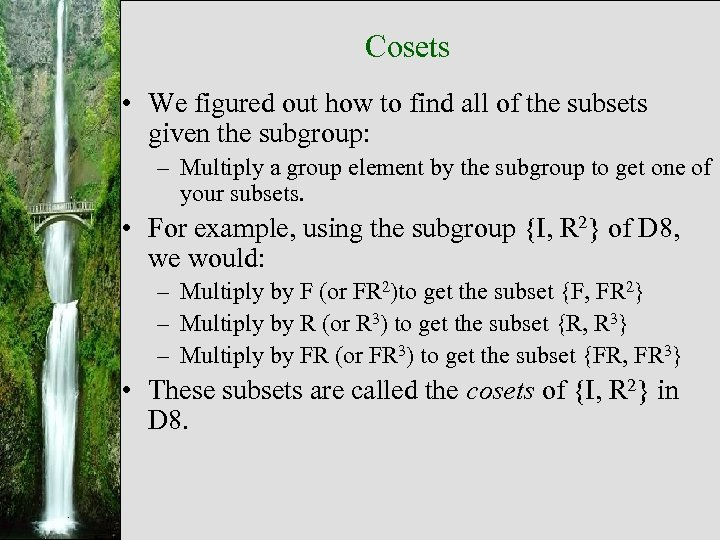Cosets • We figured out how to find all of the subsets given the subgroup: – Multiply a group element by the subgroup to get one of your subsets. • For example, using the subgroup {I, R 2} of D 8, we would: – Multiply by F (or FR 2)to get the subset {F, FR 2} – Multiply by R (or R 3) to get the subset {R, R 3} – Multiply by FR (or FR 3) to get the subset {FR, FR 3} • These subsets are called the cosets of {I, R 2} in D 8.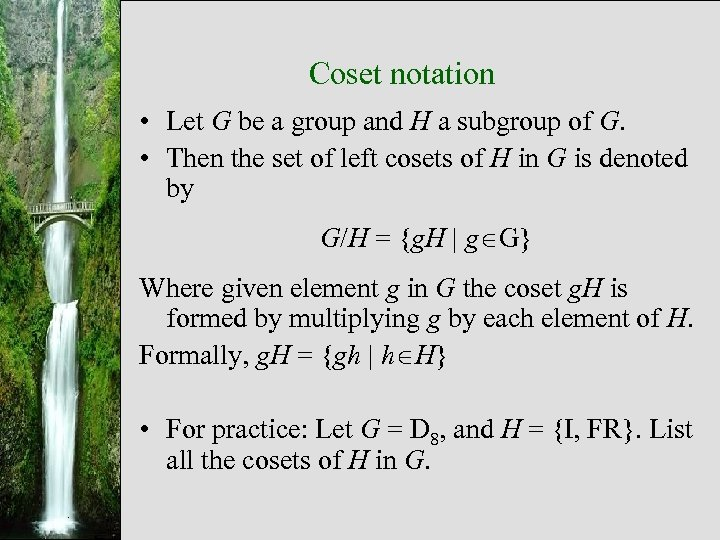Coset notation • Let G be a group and H a subgroup of G. • Then the set of left cosets of H in G is denoted by G/H = {g. H g G} Where given element g in G the coset g. H is formed by multiplying g by each element of H. Formally, g. H = {gh h H} • For practice: Let G = D 8, and H = {I, FR}. List all the cosets of H in G.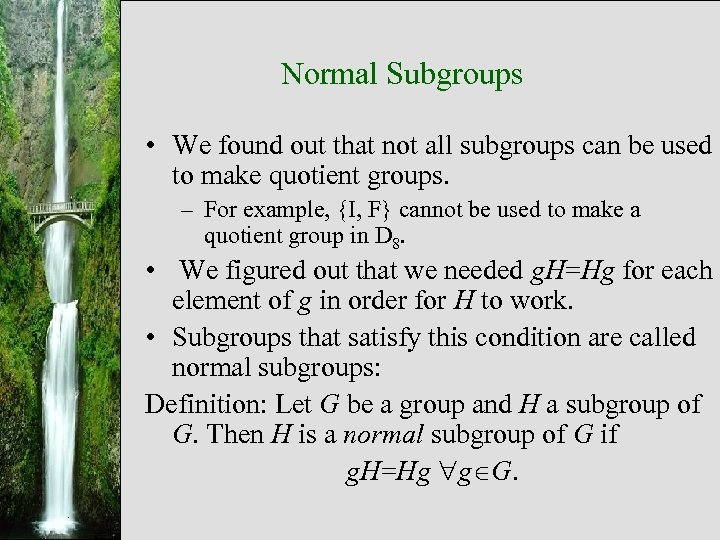Normal Subgroups • We found out that not all subgroups can be used to make quotient groups. – For example, {I, F} cannot be used to make a quotient group in D 8. • We figured out that we needed g. H=Hg for each element of g in order for H to work. • Subgroups that satisfy this condition are called normal subgroups: Definition: Let G be a group and H a subgroup of G. Then H is a normal subgroup of G if g. H=Hg g G.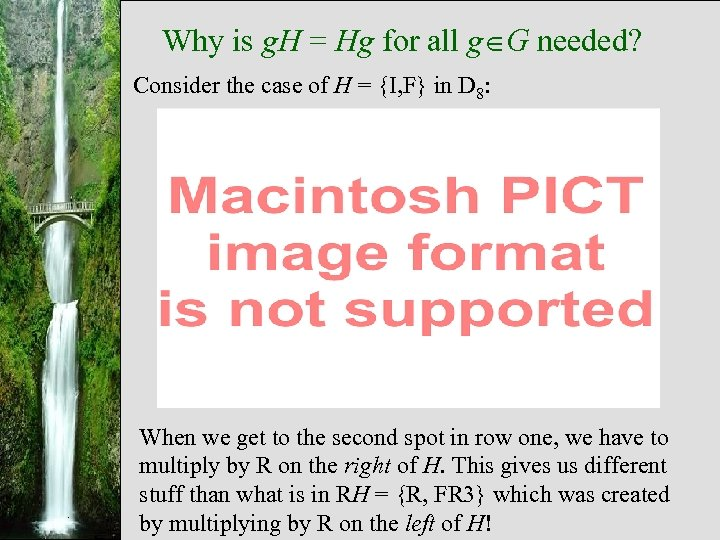Why is g. H = Hg for all g G needed? Consider the case of H = {I, F} in D 8: When we get to the second spot in row one, we have to multiply by R on the right of H. This gives us different stuff than what is in RH = {R, FR 3} which was created by multiplying by R on the left of H!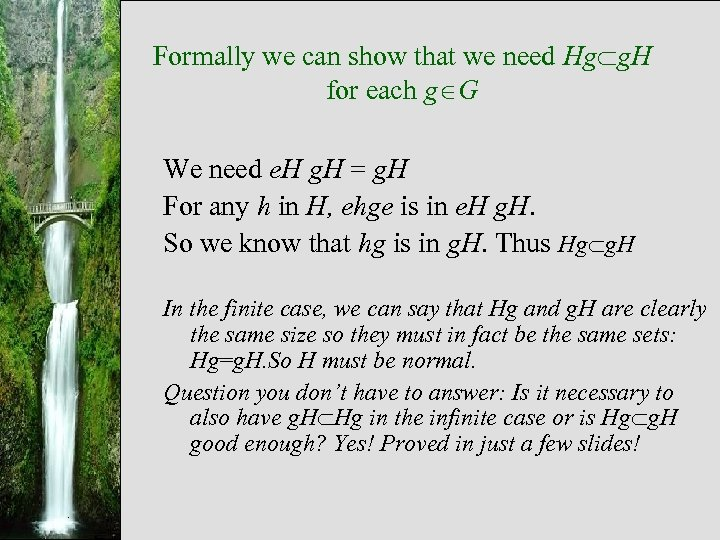Formally we can show that we need Hg g. H for each g G We need e. H g. H = g. H For any h in H, ehge is in e. H g. H. So we know that hg is in g. H. Thus Hg g. H In the finite case, we can say that Hg and g. H are clearly the same size so they must in fact be the same sets: Hg=g. H. So H must be normal. Question you don’t have to answer: Is it necessary to also have g. H Hg in the infinite case or is Hg g. H good enough? Yes! Proved in just a few slides!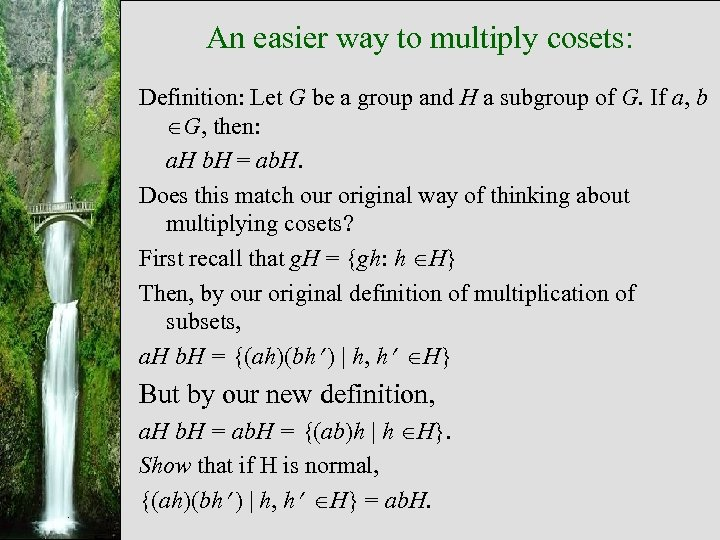An easier way to multiply cosets: Definition: Let G be a group and H a subgroup of G. If a, b G, then: a. H b. H = ab. H. Does this match our original way of thinking about multiplying cosets? First recall that g. H = {gh: h H} Then, by our original definition of multiplication of subsets, a. H b. H = {(ah)(bh ) h, h H} But by our new definition, a. H b. H = ab. H = {(ab)h h H}. Show that if H is normal, {(ah)(bh ) h, h H} = ab. H.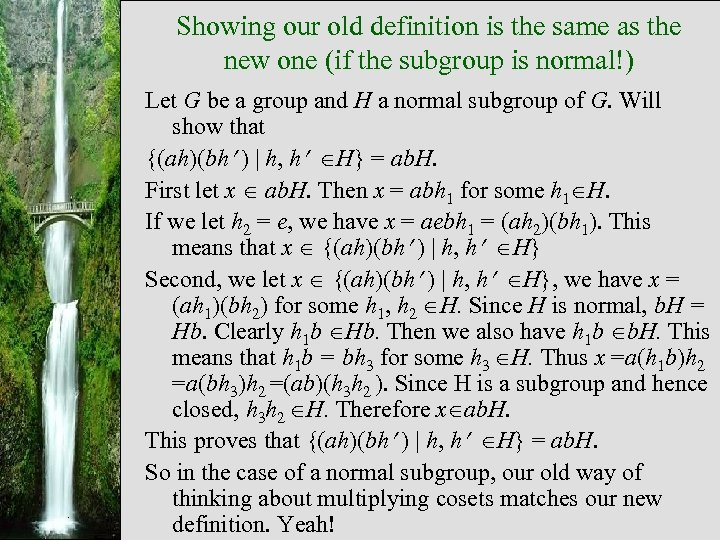Showing our old definition is the same as the new one (if the subgroup is normal!) Let G be a group and H a normal subgroup of G. Will show that {(ah)(bh ) h, h H} = ab. H. First let x ab. H. Then x = abh 1 for some h 1 H. If we let h 2 = e, we have x = aebh 1 = (ah 2)(bh 1). This means that x {(ah)(bh ) h, h H} Second, we let x {(ah)(bh ) h, h H}, we have x = (ah 1)(bh 2) for some h 1, h 2 H. Since H is normal, b. H = Hb. Clearly h 1 b Hb. Then we also have h 1 b b. H. This means that h 1 b = bh 3 for some h 3 H. Thus x =a(h 1 b)h 2 =a(bh 3)h 2 =(ab)(h 3 h 2 ). Since H is a subgroup and hence closed, h 3 h 2 H. Therefore x ab. H. This proves that {(ah)(bh ) h, h H} = ab. H. So in the case of a normal subgroup, our old way of thinking about multiplying cosets matches our new definition. Yeah!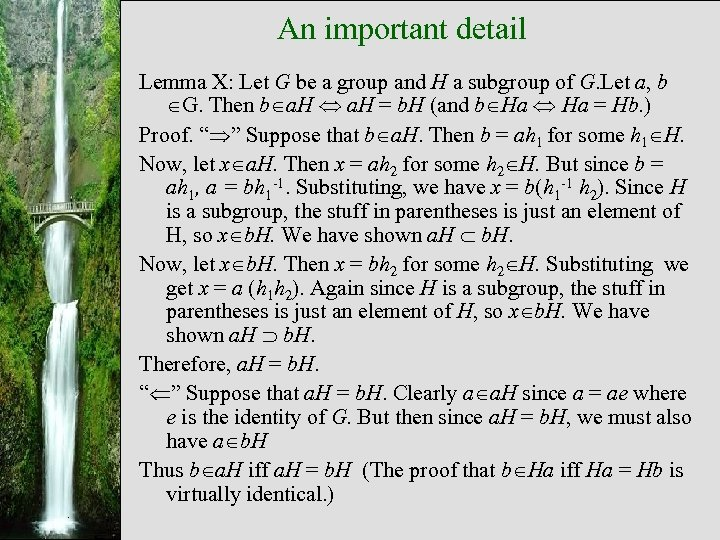An important detail Lemma X: Let G be a group and H a subgroup of G. Let a, b G. Then b a. H = b. H (and b Ha = Hb. ) Proof. “ ” Suppose that b a. H. Then b = ah 1 for some h 1 H. Now, let x a. H. Then x = ah 2 for some h 2 H. But since b = ah 1, a = bh 1 -1. Substituting, we have x = b(h 1 -1 h 2). Since H is a subgroup, the stuff in parentheses is just an element of H, so x b. H. We have shown a. H b. H. Now, let x b. H. Then x = bh 2 for some h 2 H. Substituting we get x = a (h 1 h 2). Again since H is a subgroup, the stuff in parentheses is just an element of H, so x b. H. We have shown a. H b. H. Therefore, a. H = b. H. “ ” Suppose that a. H = b. H. Clearly a a. H since a = ae where e is the identity of G. But then since a. H = b. H, we must also have a b. H Thus b a. H iff a. H = b. H (The proof that b Ha iff Ha = Hb is virtually identical. )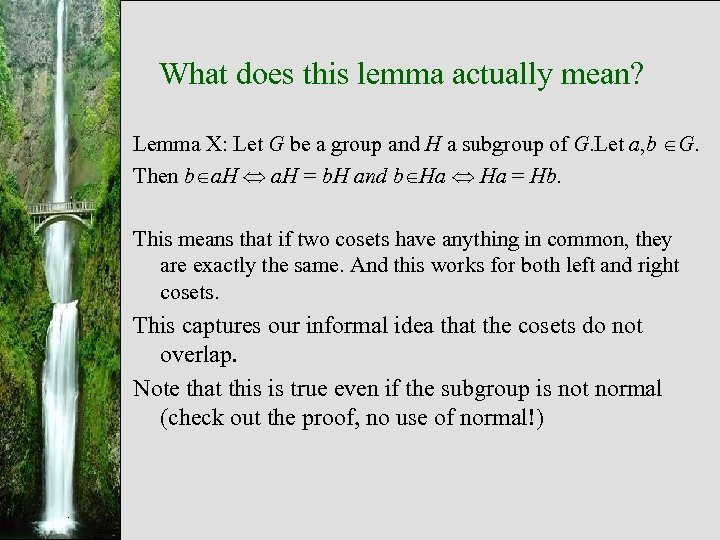What does this lemma actually mean? Lemma X: Let G be a group and H a subgroup of G. Let a, b G. Then b a. H = b. H and b Ha = Hb. This means that if two cosets have anything in common, they are exactly the same. And this works for both left and right cosets. This captures our informal idea that the cosets do not overlap. Note that this is true even if the subgroup is not normal (check out the proof, no use of normal!)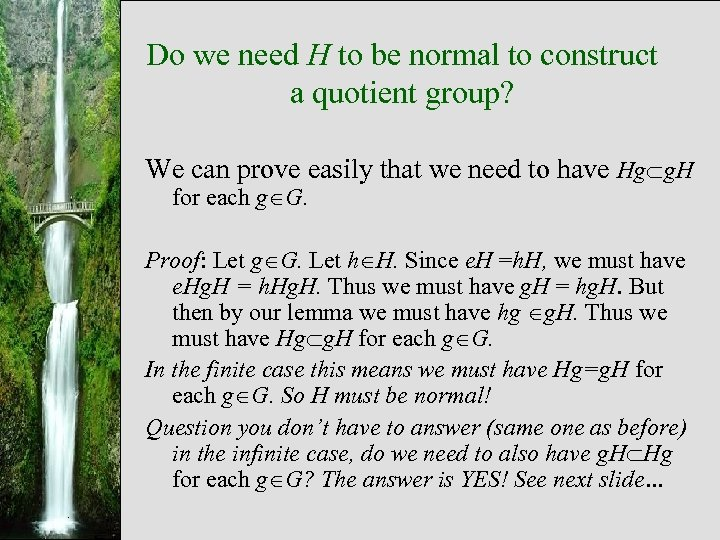Do we need H to be normal to construct a quotient group? We can prove easily that we need to have Hg g. H for each g G. Proof: Let g G. Let h H. Since e. H =h. H, we must have e. Hg. H = h. Hg. H. Thus we must have g. H = hg. H. But then by our lemma we must have hg g. H. Thus we must have Hg g. H for each g G. In the finite case this means we must have Hg=g. H for each g G. So H must be normal! Question you don’t have to answer (same one as before) in the infinite case, do we need to also have g. H Hg for each g G? The answer is YES! See next slide…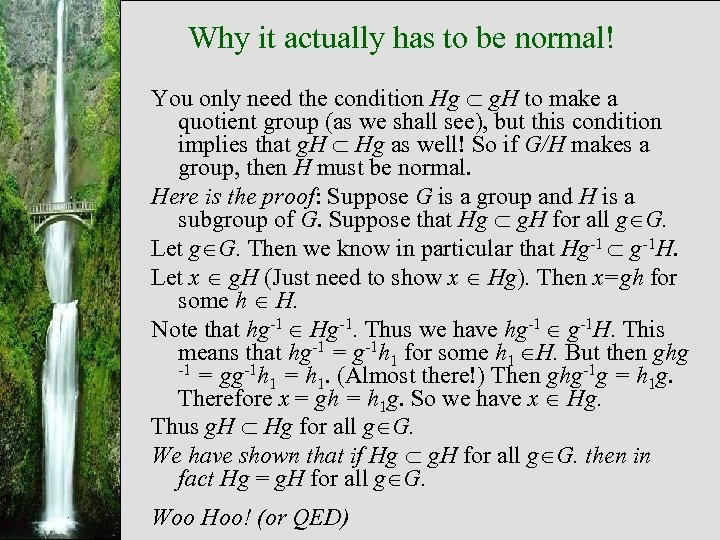Why it actually has to be normal! You only need the condition Hg g. H to make a quotient group (as we shall see), but this condition implies that g. H Hg as well! So if G/H makes a group, then H must be normal. Here is the proof: Suppose G is a group and H is a subgroup of G. Suppose that Hg g. H for all g G. Let g G. Then we know in particular that Hg-1 g-1 H. Let x g. H (Just need to show x Hg). Then x=gh for some h H. Note that hg-1 Hg-1. Thus we have hg-1 g-1 H. This means that hg-1 = g-1 h 1 for some h 1 H. But then ghg -1 = gg-1 h = h. (Almost there!) Then ghg-1 g = h g. 1 1 1 Therefore x = gh = h 1 g. So we have x Hg. Thus g. H Hg for all g G. We have shown that if Hg g. H for all g G. then in fact Hg = g. H for all g G. Woo Hoo! (or QED)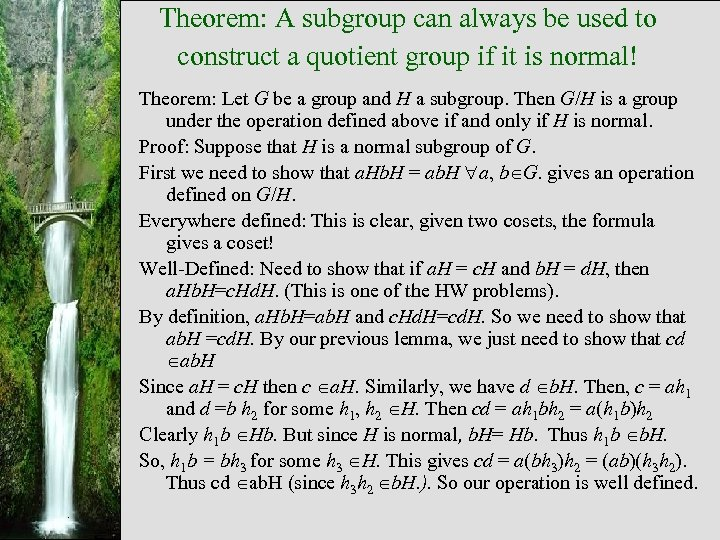Theorem: A subgroup can always be used to construct a quotient group if it is normal! Theorem: Let G be a group and H a subgroup. Then G/H is a group under the operation defined above if and only if H is normal. Proof: Suppose that H is a normal subgroup of G. First we need to show that a. Hb. H = ab. H a, b G. gives an operation defined on G/H. Everywhere defined: This is clear, given two cosets, the formula gives a coset! Well-Defined: Need to show that if a. H = c. H and b. H = d. H, then a. Hb. H=c. Hd. H. (This is one of the HW problems). By definition, a. Hb. H=ab. H and c. Hd. H=cd. H. So we need to show that ab. H =cd. H. By our previous lemma, we just need to show that cd ab. H Since a. H = c. H then c a. H. Similarly, we have d b. H. Then, c = ah 1 and d =b h 2 for some h 1, h 2 H. Then cd = ah 1 bh 2 = a(h 1 b)h 2 Clearly h 1 b Hb. But since H is normal, b. H= Hb. Thus h 1 b b. H. So, h 1 b = bh 3 for some h 3 H. This gives cd = a(bh 3)h 2 = (ab)(h 3 h 2). Thus cd ab. H (since h 3 h 2 b. H. ). So our operation is well defined.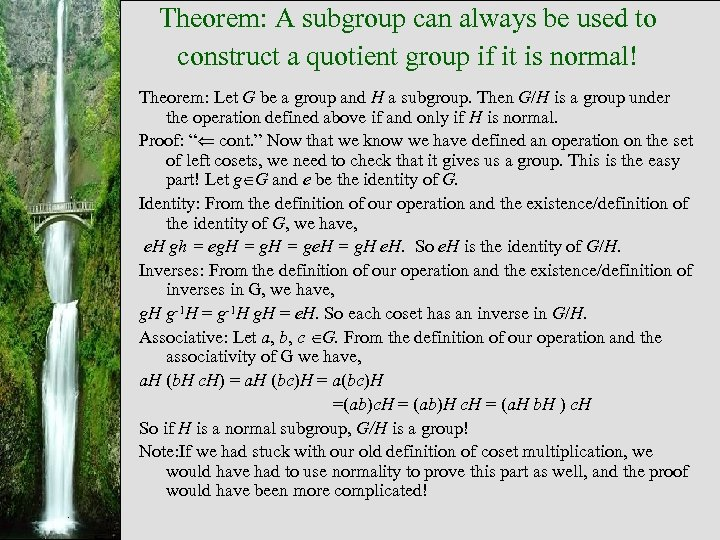Theorem: A subgroup can always be used to construct a quotient group if it is normal! Theorem: Let G be a group and H a subgroup. Then G/H is a group under the operation defined above if and only if H is normal. Proof: “ cont. ” Now that we know we have defined an operation on the set of left cosets, we need to check that it gives us a group. This is the easy part! Let g G and e be the identity of G. Identity: From the definition of our operation and the existence/definition of the identity of G, we have, e. H gh = eg. H = ge. H = g. H e. H. So e. H is the identity of G/H. Inverses: From the definition of our operation and the existence/definition of inverses in G, we have, g. H g-1 H = g-1 H g. H = e. H. So each coset has an inverse in G/H. Associative: Let a, b, c G. From the definition of our operation and the associativity of G we have, a. H (b. H c. H) = a. H (bc)H = a(bc)H =(ab)c. H = (ab)H c. H = (a. H b. H ) c. H So if H is a normal subgroup, G/H is a group! Note: If we had stuck with our old definition of coset multiplication, we would have had to use normality to prove this part as well, and the proof would have been more complicated!